Select Page

# CBSE Solutions for MCQ 12 Science Maths Application of Derivatives in English

CBSE Solutions for MCQ 12 Science Maths Application of Derivatives in English to enable students to get Solutions in a narrative video format for the specific question.

Expert Teacher provides CBSE Solutions for MCQ 12 Science Maths Application of Derivatives through Video Solutions in English language. This video solution will be useful for students to understand how to write an answer in exam in order to score more marks. This teacher uses a narrative style for a question from Application of Derivatives not only to explain the proper method of answering question, but deriving right answer too.

Please find the question below and view the Solution in a narrative video format.

Question:

Solution Video in English:

You can select video Solutions from other languages also. Please check Solutions in ( Hindi )

## Similar Questions from CBSE, 12th Science, Maths, Application of Derivatives

Question 1 : The volume of a cube is increasing at the rate of 9 cubic centimetres per second. How fast is the surface area increasing when the length of an edge is 10 centimetres? (View Answer Video)

Question 2 : The rate of change of the area of a circle with respect to its radius r at r=6cm is: (View Answer Video)

Question 3 : The total cost C(x) (in Rs) associated with the production of 'x' units of an item is given by :
C (x)=0.005x3-0.02x2+30x+5000.
Find the marginal cost when 3 units are produced, where by marginal cost we mean the instantaneous rate of change of total cost at any level of output. (View Answer Video)

Question 4 : For all real values of x, the minimum value of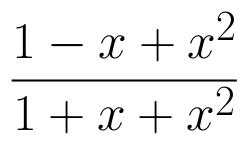is  (View Answer Video)

Question 5 : For the curve, y=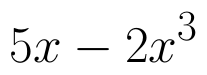if x increases at the rate of 2 units/sec, then how fast is the slope of curve changing when x=3? (View Answer Video)

### Three Dimensional Geometry

Question 1 : Find the distance between the point (7, 2, 4) and the plane determined by the points A(2, 5, -3), B(-2, -3, 5) and C(5, 3, -3). (View Answer Video)

Question 2 : Find the vector equation of a plane which is at a distance of 5 units from the origin and its normal vector is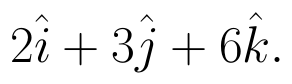(View Answer Video)

Question 3 : Find the equation of the plane that contains the point (1, -1, 2) and is perpendicular to both the planes 2x + 3y - 2z = 5 and x + 2y - 3z = 8. Hence find the distance of point P(-2, 5, 5) from the plane obtained above. (View Answer Video)

Question 4 : Find the distance between the point (5, 4, -6) and its image in xy-plane. (View Answer Video)

Question 5 : The cartesian equation of a line is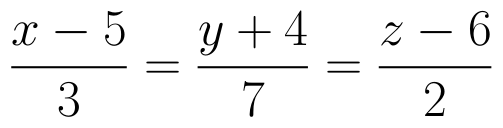, write its vector form. (View Answer Video)

### Linear Programming

Question 1 : The objective function is maximum or minimum, which lies on the boundary of the feasible region. (View Answer Video)

### Inverse Trigonometric Functions

Question 1 :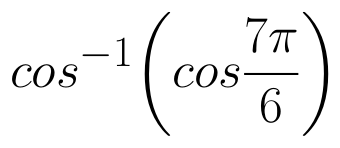is equal to :
Question 2 :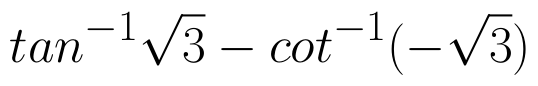is equal to : (View Answer Video)
Question 3 : Write the principal value of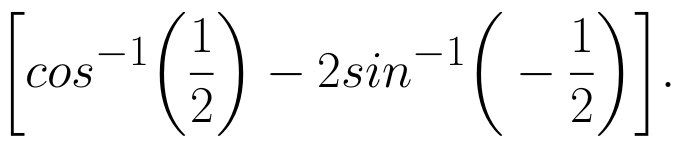(View Answer Video)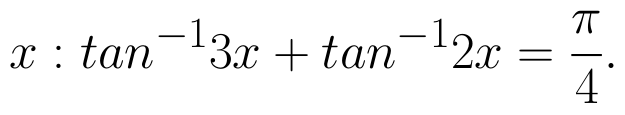(View Answer Video)
Question 5 : Write the value of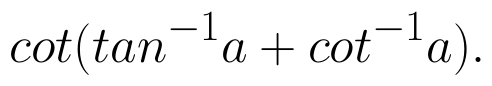(View Answer Video)